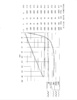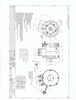# ME1115

## Product Description

Output Power of 12 KW Continuous, 30 KW Peak (at 96 volts)

Designed for long life. No brush maintenance. The motor is 92% efficient at voltages between 24 to 96 VDC. Continuous current of 150 Amps. This is a 3-phase, Y-connected Permanent Magnet Synchronous Motor with an axial air gap and Sine/Cosine Speed Sensor. It has two stators with a rotor in the center.

1) This is a 4 pole motor (8 magnets). 2) The Phase to Phase winding resistance is 0.013 Ohms. 3) The maximum recommended rotor speed is 5000 RPM. 4) Voltages from 0 to 96 VDC input to the control. 5) Torque constant of 0.15 Nm per Amp 6) The Inductance Phase to Phase is 0.10 Milli-Henry with a 28 turns per phase. 7) Armature Inertia is 45 Kg Cm Squared. 8) Continuous current of 150 Amps. 9) Peak current of 420 Amps AC for 1 minute. 10) Weight of 34 pounds. 11) Peak Stall Torque if 60 ft lb. 12) This is an Open Frame, Fan Cooled motor.

Includes temperature sensor.MR1115 Dynamometer PlotDrawing ME1115 Rev. A